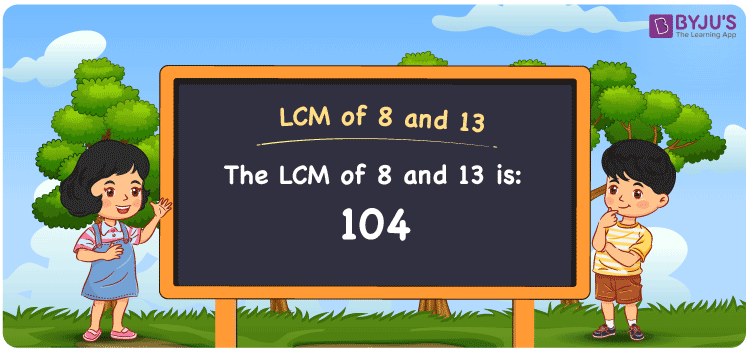Checkout JEE MAINS 2022 Question Paper Analysis : Checkout JEE MAINS 2022 Question Paper Analysis :

# LCM of 8 and 13

LCM of 8 and 13 is 104. LCM is the number which is evenly divisible by 8 and 13. From the common multiples, the least common multiple of 8 and 13 can be calculated. The multiples of 8 are (8, 16, 24, 32, 40, 48, ….) and the multiples of 13 are (13, 26, 39, 52, 65, ….) respectively. Division, Prime factorisation and listing of the multiples are the most common methods used to get the LCM value.

## What is LCM of 8 and 13?

The answer to this question is 104. The LCM of 8 and 13 using various methods is shown in this article for your reference. The LCM of two non-zero integers, 8 and 13, is the smallest positive integer 104 which is divisible by both 8 and 13 with no remainder.## How to Find LCM of 8 and 13?

LCM of 8 and 13 can be found using three methods:

• Prime Factorisation
• Division method
• Listing the multiples

### LCM of 8 and 13 Using Prime Factorisation Method

The prime factorisation of 8 and 13, respectively, is given by:

8 = 2 x 2 x 2 = 2³

13 = 13¹

LCM (8, 13) = 104

### LCM of 8 and 13 Using Division Method

We’ll divide the numbers (8, 13) by their prime factors to get the LCM of 8 and 13 using the division method (preferably common). The LCM of 8 and 13 is calculated by multiplying these divisors.

 2 8 13 2 4 13 2 2 13 13 1 13 x 1 1

No further division can be done.

Hence, LCM (8, 13) = 104

### LCM of 8 and 13 Using Listing the Multiples

To calculate the LCM of 8 and 13 by listing out the common multiples, list the multiples as shown below

 Multiples of 8 Multiples of 13 8 13 16 26 24 39 32 52 40 65 48 78 56 91 64 104 72 117 80 130 88 143 96 156 104 169 112 182

LCM (8, 13) = 104

## Video Lesson on Applications of LCM## LCM of 8 and 13 Solved Examples

If the product of two numbers is 104, calculate the LCM if the GCD is 1.

Solution:

It is given that

GCD = 1

Product of two numbers = 104

We know that

LCM x GCD = product of two numbers

LCM = product of two numbers/ GCD

LCM = 104/1

LCM = 104

Hence, the LCM is 104.

## Frequently Asked Questions on LCM of 8 and 13

### What is the LCM of 8 and 13?

We know that,

Multiples of 8 = 8, 16, 24, 32, 40, 48, ….

Multiples of 13 = 13, 26, 39, 52, 65, ….

The smallest multiple exactly divisible by 8 and 13 is 104.

### If the LCM of 8 and 13 is 104, determine the GCF.

LCM x GCF = 8 x 13

Given,

LCM of 8 and 13 is 104

104 x GCF = 104

GCF = 104/104 = 1

### What are the methods used to find the LCM of 8 and 13?

The methods used to find the LCM of 8 and 13 are

Division method

Prime Factorisation

Listing the multiples